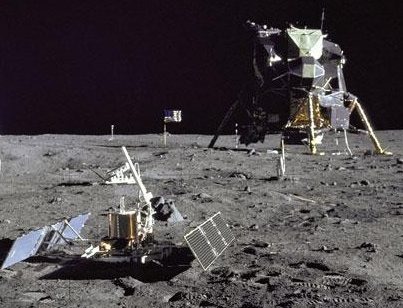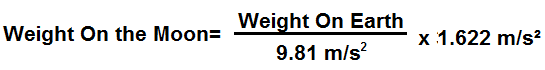﻿ Weight On the Moon Calculator ﻿# Weight on the Moon Calculator### How Much Would You Or Any Object Weigh On the Moon?Enter Weight: Any unit grams (g) kilograms (kg) pounds (lbs)

This Weight on the Moon Conversion Calculator calculates your weight or the weight that any object would be if on the moon.

To use this calculator, a user just enters his or her weight and clicks the 'Calculate' button, and his/her weight will automatically be computed and shown below. The weight can be entered in any units and the resultant answer will be in the same units which the user has input. Thus, for example, if a user enters 200lbs, the resultant answer of the weight on the moon would be 33.07lbs. The units will always match. Just for the sake of clarity, a user can select the units he wants the answer to appear in, whether grams, kilograms, or pounds, and that unit will show in the answer.

This calculates the weight on the moon from the weight of the object on earth. Being that it takes the weight of an object on earth and converts it to lunar weight, the formula is Weight on the Moon= (Weight on Earth/9.81m/s2) * 1.622m/s2. To find the weight on the moon, we divide the weight on earth by the earth's force of gravity, which is 9.81m/s2. This calculates the mass of the object. Once we have the object's mass, we can find the weight by multiplying it by the gravitational force, which it is subject to. Being that the moon has a gravitational force of 1.622m/s2, we multiply the object's mass by this quanitity to calculate an object's weight on the moon.

So an object or person on the moon would weigh 16.5% its weight on earth. Therefore, a person would be much lighter on the moon. Conversely, a person is 83.5% heavier on earth than on the moon.

What causes the differences in weight between the various planets? The answer is the gravitational force which a planet is subject to. Earth has much more mass than the moon. Therefore, the sun pulls on it with greater force, because of the greater mass. You probably are familiar that gravity is a downward force. It pushes you back to the Earth's surface. This is why when you jump, you come back down. Being that the earth has greater gravitational force, it pulls object downward with greater force. This translates into greater weight.

If you want to calculate the weight of all of the planets simultaneously, see our Weight on Planets Calculator.

Related Resources

﻿